# Stochastic process with stationary increments

(diff) ← Older revision | Latest revision (diff) | Newer revision → (diff)

A stochastic process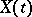in discrete or continuous timesuch that the statistical characteristics of its increments of some fixed order do not vary with time (that is, are invariant with respect to the time shifts). As in the case of stationary stochastic processes (cf. Stationary stochastic process), one distinguishes two types of such processes, namely stochastic processes with stationary increments in the strict sense, for which all finite-dimensional probability distributions of increments ofof a given order at the pointsand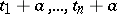for anycoincide with one another, and stochastic processes with stationary increments in the wide sense, for which the mean values of an increment atand the second moments of the increments atand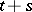do not depend on.

In the case of processesin discrete timeone can always pass from the consideration ofto that of the new stochastic process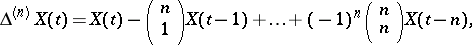where the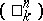are binomial coefficients. Ifis a stochastic process with stationary increments of order, then the processis stationary in the usual sense. Thus, in the case of discrete time, the theory of stochastic processes with stationary increments reduces easily to that of the more particular stationary stochastic processes. However, from the point of view of applications, the use of the concept of a stochastic process with stationary increments and discrete timeoften turns out to be very convenient, since for many explicit non-stationary time series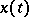,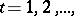met in practice, the series of their incrementsof some ordercan be regarded as realizations of a stationary stochastic process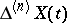. In particular, G. Box and G. Jenkins showed in  that, when solving many practical problems, real time series can often be regarded as realizations of a so-called auto-regressive process, an integrated moving-average process that represents a special stochastic process with stationary increments and discrete time (see also ).

Examples of stochastic processes with stationary increments of the first order (in the strict sense) and in continuous timeare a Wiener process and a Poisson process. Both of these also belong to the narrower class of processes with independent increments of the first order. In the case of continuous, the theory of stochastic processes with stationary increments does not reduce directly to the theory of the simpler stationary processes. The correlation theory of stochastic processes with stationary increments of the first order (that is, the theory of the corresponding processes in the wide sense), was developed by A.N. Kolmogorov  (see also ). An analogous theory of stochastic processes with stationary increments of order, whereis an arbitrary positive integer, was considered in . A central position in the correlation theory of stochastic processes with stationary increments is occupied by the derivation of the spectral decomposition of such processes and of their second-order moments. The concept of a generalized stochastic process (cf. Stochastic process, generalized) can be used to simplify the theory of stochastic processes with stationary increments. Since in the theory of generalized stochastic processes, any stochastic processhas derivatives of all orders (which are again generalized stochastic processes), a stochastic process with stationary increments of ordercan be defined as a stochastic processwhose-th derivativeis an (in general, generalized) stationary stochastic process (see ).

How to Cite This Entry:
Stochastic process with stationary increments. Encyclopedia of Mathematics. URL: http://encyclopediaofmath.org/index.php?title=Stochastic_process_with_stationary_increments&oldid=12854
This article was adapted from an original article by A.M. Yaglom (originator), which appeared in Encyclopedia of Mathematics - ISBN 1402006098. See original article